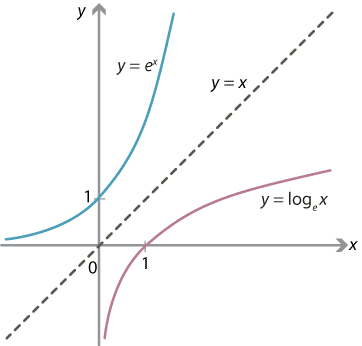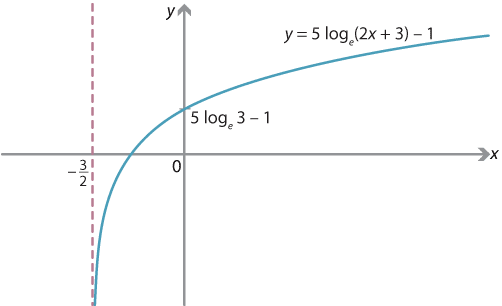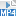## Content

### Graphing logarithmic functions

For any $$a>1$$, the functions $$f(x) = a^x$$ and $$g(x) = \log_a x$$ are inverse functions, since

$f(g(x)) = a^{\log_a x} = x$ and $g(f(x)) = \log_a (a^x) = x.$

The graphs of $$y=f(x)$$ and $$y=g(x)$$ are therefore obtained from each other by reflection in the line $$y=x$$.The symmetry between the graphs of $$y = e^x$$ and $$y = \log_e x$$.
Detailed description

The graph of the logarithmic function $$\log_a x$$, for any $$a>1$$, has the $$y$$-axis as a vertical asymptote, but has no critical points. Similarly, any other graph obtained from this graph by dilations, reflections in the axes and translations also has a vertical asymptote and no critical points.

Note. The graph of a logarithmic function does not have a horizontal asymptote. As $$x$$ becomes large positive, the graph of $$y = \log_a x$$ becomes very flat: the derivative

$\dfrac{dy}{dx} = \dfrac{1}{x \, \log_e a}$ approaches 0. Thus, $$\log_a x$$ increases very slowly. However $$\log_a x$$ still increases all the way to infinity: $\log_a x \to \infty \text{ \ as \ } x \to \infty.$

One way to see this is to look at the symmetry in the graph above. The graph $$y=a^x$$ is defined for all values of $$x$$, but when $$x$$ is large the corresponding value of $$y$$ is very large. By symmetry, $$y = \log_a x$$ takes all values of $$y$$, but when $$y$$ is large the corresponding value of $$x$$ is very large.

Exercise 17

Prove directly that $$\log_a x$$ can take an arbitrarily large value. Consider a large positive number $$N$$. Does $$\log_a x$$ ever take the value $$N$$?

Exercise 18

Show that there is a point on the graph of $$y=\log_e x$$ such that, to go one unit upwards along the graph, you have to go a million units to the right.

That is, find $$x$$ and $$y$$ such that

$y = \log_e x \qquad \text{and} \qquad y + 1 = \log_e (x + 10^6).$

#### Example

Draw the graph of $$y = 5 \,\log_e(2x+3)-1$$.

#### Solution

The equation can be written as $$y = 5 f\bigl(2(x+\dfrac{3}{2})\bigr) - 1$$, where $$f(x) = \log_e x$$. Therefore, the graph is obtained from the graph of $$y = \log_e x$$ by successively applying the following transformations:

• dilation by a factor of $$\dfrac{1}{2}$$ in the $$x$$-direction from the $$y$$-axis, which gives the graph of $$y = \log_e (2x)$$
• dilation by a factor of 5 in the $$y$$-direction from the $$x$$-axis, which gives $$y = 5 \, \log_e (2x)$$
• translation by $$\dfrac{3}{2}$$ in the negative $$x$$-direction, giving $$y = 5 \, \log_e \bigl( 2(x + \dfrac{3}{2})\bigr) = 5 \, \log_e (2x+3)$$
• translation by 1 in the negative $$y$$-direction, which gives $$y = 5 \, \log_e (2x+3) - 1$$.

These transformations produce a vertical asymptote at $$x=-\dfrac{3}{2}$$. There are no critical points.Detailed description

Exercise 19

Consider the graph $$y = \log_3 x$$. Explain why the following two transformations of the graph have the same effect:

• dilation in the $$x$$-direction from the $$y$$-axis with factor 9
• translation by 2 units down (that is, in the negative $$y$$-direction).

Screencast of exercise 19